Next: NUMERICAL MATRIZANTS Up: Mathematical physics in stratified Previous: Mathematical physics in stratified

# FROM PHYSICS TO MATHEMATICS

First Step:

The first step is to write down all the basic partial differential equations of classical physics which relate to the problem of interest. Do not write down equations containing second space derivatives which are derived from first-derivative equations. Write down the first-derivative equations. Write each component of vector or matrix equations.

In acoustics we have the gradient pressure p gives rise to an acceleration of mass density.For convenience we restrict motion to the x, z plane. Letting u and w represent x and z components of velocity we have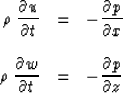(1) (2)
Another equation which is important in acoustics is the one that states that the divergence of velocity multiplied by the incompressibility K yields the rate of pressure decrease.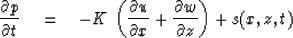(3)
In (9-1-3) we included a pressure source s. This is something to be externally prescribed. The quantity s may be a source of chemical energy such as an explosion; thus it may vanish everywhere except at a point. Distributed sources are also often of interest; for example, radioactive rocks in a heat-flow model of the earth. To be more general, we could also have put momentum sources into (9-1-1) or (9-1-2), but the basic principles will be adequately exemplified with a source only in (9-1-3).

Second step:

The wave disturbance variables are taken to be unknown and the material properties known. Count equations and unknowns. We have three equations; u, w, and p are the three unknowns. We take K,, and s to be known. Notice that the equations are linear in the unknowns. Now we make the stratification assumption; that is, we assume K andare functions of depth z only and that they are constant in x. Since our linear equations now have constant coefficients with respect to x and t, we may always expect sinusoidal solutions in x and t. We do not know what to expect of our solutions in the z coordinate because of the arbitrary z-dependence of the coefficients K and.This leads to step three.

Third step:

Fourier transform time and the space coordinates with constant coefficients. In other words, we make the following substitution into (9-1-1), (9-1-2), and (9-1-3)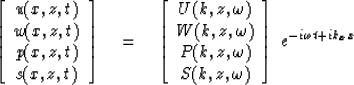(4)

After substitution, cancel the exponential and obtain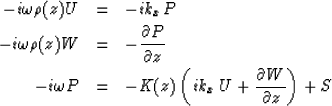(1) (2) (3)

Fourth step:

Eliminate algebraically the algebraic unknowns. In other words, when you examine (9-1-5) you see terms in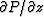but you do not see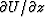.This means that U is an algebraic variable which can be eliminated by purely algebraic means. We do this by substituting (9-1-5a), into (9-1-5c).

Fifth step:

Bring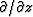terms to the left, bring all others to the right, and arrange terms into a neat matrix form. We have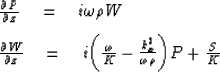and then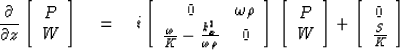(6)

Sixth step:

Recognize that, no matter the physical problem with which you started, you should have a matrix first-order differential equation of the form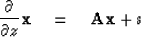(7)
where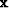is a vector containing the field variables of interest,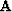is a matrix depending on temporal and spatial frequency and on material properties, andis a (possibly absent) vector function of the sources.

Before we look into techniques of solving (9-1-7) we can immediately deduce that in a source-free region the field variablesare smoother functions than the material properties. To see this, consider two homogeneous layers in contact. At the contact thematrix has step-function discontinuities. Now let us see whether the wave fields incan have step-function discontinuities. Obviously they cannot, since a step discontinuity inwould imply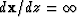,whereas (9-1-7) in a source-free region states that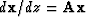and bothandare supposed finite. This does not mean that all field variables are always smooth. The algebraic variables eliminated in the fourth step can and often will be discontinuous at layer boundaries.

## EXERCISES:

1. What form does (9-1-7) take for the heat-flow equation? Include radioactive sources. [HINT: See equations (10-1-1) and (10-1-2).]
2. Using Maxwell's equations,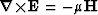,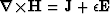,and Ohm's law,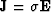where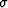is conductivity, set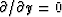and derive (9-1-7).
3. In electrostatics the electric field in the ionosphere may be derived from a potential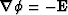, the divergence of electrical current vanishes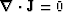and Ohm's law must have an extra term due to wind (a current source due to differential drag on ions and electrons across the earth's magnetic field)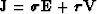.Assume you know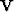.What form does (9-1-7) take assumingand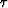to be scalars? Indicate how the calculation proceeds if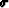and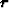are matrices (assume you have the inverse of any matrix you wish).
4. In magnetostatics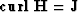and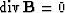, and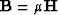.Takingas given, what is the form of (9-1-7)?
5. This exercise illustrates the linearization of nonlinear problems. For acoustic waves in a stratified windy atmosphere we used the trial solutions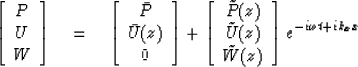Reduce the partial differential equations to a matrix ordinary differential equation. HINT: The horizontal acceleration terms is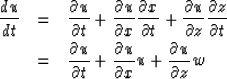with a like term for vertical acceleration. Drop second-order terms in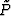,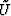, and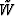.
6. Two equations come from heat flow: (Hx, Hz) equals the conductivitymultiplied by the negative of the temperature gradient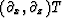.The time derivative of temperature multiplied by the heat capacity c equals the negative of the heat-flow divergence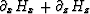gives another equation. Insert the trial solutions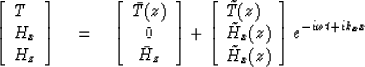(a)
First derive steady-state equations for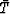and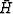assuming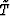and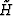vanish.
(b)
Assumingandsatisfy part (a), find equations forand.

(c)
Repeat (a) and (b) assuming linear temperature dependence of heat capacity and conductivity, i.e.,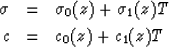You will have to drop squared terms inand.
7. Consider a compressible liquid sphere pulsating radially under its own gravitational attraction. What is the form of (9-1-6)?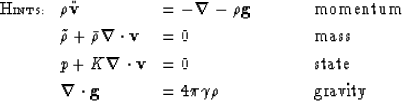Next: NUMERICAL MATRIZANTS Up: Mathematical physics in stratified Previous: Mathematical physics in stratified
Stanford Exploration Project
10/30/1997